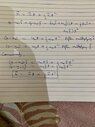# A particle motion problem in the x-y plane with constant acceleration

• rudransh verma
In summary, at t=0, x=0, the object has a x-velocity of 7m/s and an x-acceleration of -9m/s². If the maximum x-coordinate reached is X, then the x-velocity is v=7i^ m/s when x=X, and the time at which x=X is t=0. The value of X is 2.7222...m.
haruspex said:
Sure.
To get the scalar eqns from the vector, take the dot product with, respectively, ##\hat x, \hat y##.
Conversely, multiply the scalar equns by respectively, ##\hat x, \hat y##, and add them to produce the vector equn.
Still working on latex

#### Attachments

•image.jpg
47 KB · Views: 62
rudransh verma said:
Still working on latex
Yes.